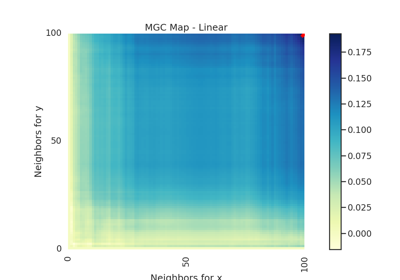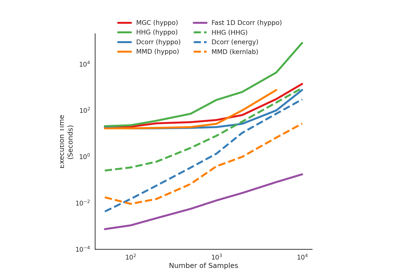# linear¶

hyppo.tools.linear(n, p, noise=False, low=- 1, high=1)

Linear simulation.

Linear $$(X, Y) \in \mathbb{R}^p \times \mathbb{R}$$:

$\begin{split}X &\sim \mathcal{U}(-1, 1)^p \\ Y &= w^T X + \kappa \epsilon\end{split}$
Parameters
Returns

x,y (ndarray) -- Simulated data matrices. x and y have shapes (n, p) and (n, 1) where n is the number of samples and p is the number of dimensions.

## Examples using hyppo.tools.linear`¶